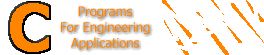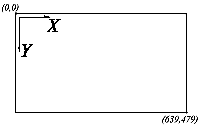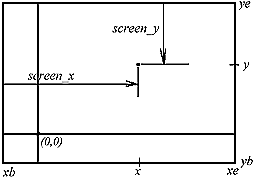Plotting graphs and charts When we need to plot a graph of any given function, we face the problem of interfacing the computer coordinate system. The figure below shows the computer coordinate system at a resolution of 640x480.As shown in the figure, the direction of the positive Y-axis is opposite to the one used in engineering charts. So, we have to consider this when rescaling and translating the axes. Almost all monitors connected to PCs today support the VGA resolution of 640x480x16, which is supported by Borland and Turbo C, and most other C compilers. So, we will use this mode in all our examples. We will use a function screen_x() that returns the actual screen X-coordinate given the begining and end X-coordinates, xb and xe resp., and the desired X-coordinate. The relation between all these is shown in the figure below.Another function, screen_y() does the same on Y-coordinates.Here is the ready-to-use code for both functions: #define MAXX 640 #define MAXY 480 float screen_x(float, float, float); float screen_y(float, float, float); float screen_x(float xb, float x, float xe) { return ((x-xb)/(xe-xb)*MAXX); } float screen_y(float yb, float y, float ye) { return (MAXY-(y-yb)/(ye-yb)*MAXY); } Usage of both of the above functions is illustrated by the following examples: Plotting the quadratic function y=ax2+bx+c Zooming and panning-graphs in action! Plotting data from a file